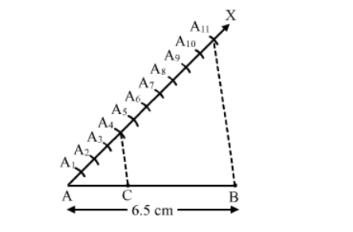# Draw a line segment AB of length 6.5 cm and divide it in the ratio 4 : 7.`
Question:

Draw a line segment AB of length 6.5 cm and divide it in the ratio 4 : 7. Measure each of the two parts.

Solution:

Steps of Construction :

Step 1 . Draw a line segment AB = 6.5 cm.

Step 2. Draw a ray $A X$, making an acute angle $\angle B A X$.

Step 3. Along AX, mark (4+7) =11 points A1, A2, A3A4, A5, A6, A7, A8, A9, A10, A11,  such that

AA1 = A1A2 = A2A3 = A3A4 = A4A5 = A5A6 = A6A7 = A7A8 = A8A9 = A9A10 = A10A11

Step 4. Join A11B.
Step 5. From A4draw A4">A11B, meeting AB at C.

Thus, C is the point on AB, which divides it in the ratio 4:7.Thus, AC : CB = 4:7
From the figure, AC = 2.36 cm
CB = 4.14 cm